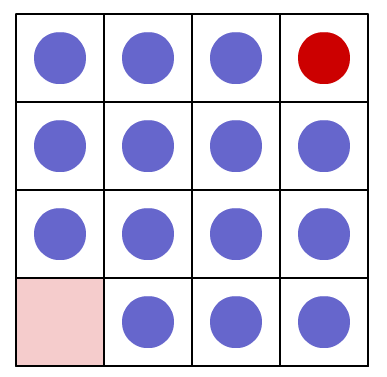#### You may also like### Just Rolling Round

P is a point on the circumference of a circle radius r which rolls, without slipping, inside a circle of radius 2r. What is the locus of P?### Coke Machine

The coke machine in college takes 50 pence pieces. It also takes a certain foreign coin of traditional design...### Just Opposite

A and C are the opposite vertices of a square ABCD, and have coordinates (a,b) and (c,d), respectively. What are the coordinates of the vertices B and D? What is the area of the square?

# Changing Places

##### Age 14 to 16Challenge Level

A four by four square grid contains fifteen counters with the bottom left hand square empty. The counter in the top right hand square is red and the rest are blue. The aim is to slide the red counter from its starting position to the bottom left hand corner in the least number of moves. You may only slide a counter into an empty square by moving it up, down, left or right but not diagonally.Explore a four by four array. How many moves did you take to move the red counter to HOME (pink square)?

Can you do it in fewer moves? What is the least number of moves you can do it in?

Try a smaller array. How many moves did you take to move the red counter to HOME?
Try a larger array. What is the least number of moves you can do it in?

Have you a strategy for moving down each array?

On which move does the red counter make its first move?

On which moves does the red counter make its other moves?
Can you predict the number of moves that the red counter makes on the way HOME?
Why is the least number of moves ALWAYS odd?
Can you predict what the least number of moves will be for any square array?

Can you explain why YOUR rule works?